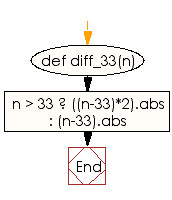﻿ Ruby Basic exercises: Compute the absolute difference between n and 33 - w3resource# Ruby Basic Exercises: Compute the absolute difference between n and 33

## Ruby Basic: Exercise-17 with Solution

Write a Ruby program to compute the absolute difference between n and 33 and return double the absolute difference if n is over 33.

Ruby Code:

``````def diff_33(n)
n > 33 ? ((n-33)*2).abs : (n-33).abs
end
print diff_33(47),"\n"
print diff_33(17)
``````

Output:

```28
16
```

Flowchart:Ruby Code Editor: